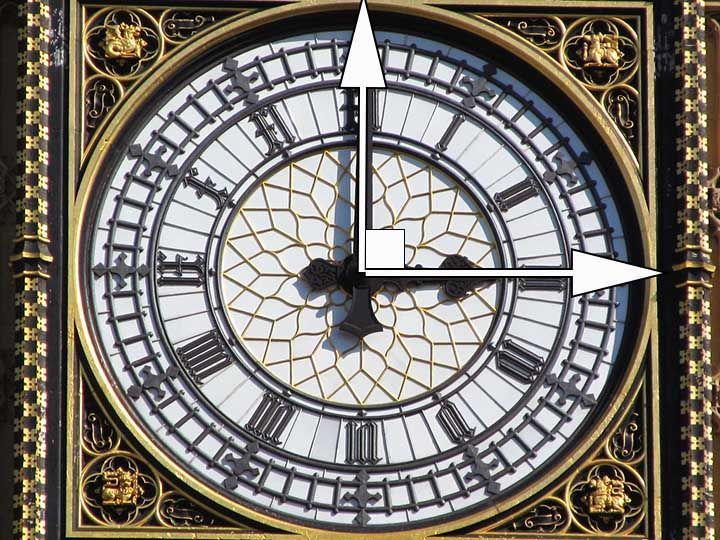# Simply Interesting

Simple Interest is calculated by the formula

S.I. = (P x R x T)/100.

where P = Principal, R = Rate of Interest and T = Period of Interest.

# Find the Time

The cost of construction of the panels is \$625000

The founder of the project took a loan.He was charged interest at 12.5% per annum.

The interest paid by the founder was equal to the principal.

Find the number of years for which the loan was taken.

Solution

T = S.I. x 100/PR =

\$625000 x 100/ \$625000 x 12.5 = 100/12.5 =

8 years.

# Find the Rate

The cost of construction of the panels is \$625000

The founder of the project took a loan.The duration of the loan was 16 years.

The interest paid by the founder was equal to the principal.

Find the interest rate at which he took the loan.

Solution

R = S.I. x 100/PT =

\$625000 x 100/ \$625000 x 16 = 100/16 =

6.25%

# Access All Content

## More from Grade 6 Math# Types of Lines

All you need to know about lines, parallel, perpendicular and intersecting lines. Along with all the examples from the world around you.# Types of Angles

Learn about the different types of angles acute, right, obtuse and more through exciting facts from the Wonders of the World.# Celsius to Fahrenheit

Learn how to check the temperature, anytime and anywhere.